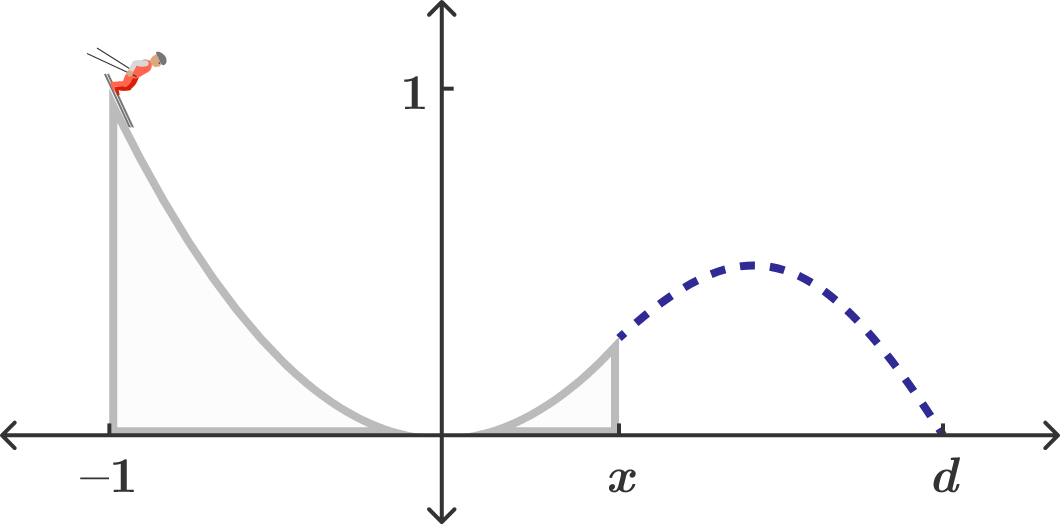# Launching from a Cut ParabolaYou're building a ski jump for the upcoming Winter Olympics and it's been decided that the hill and ramp will be formed from the shape of a parabola, so that at any $x,$ the height is given by $h(x) = x^2.$

If the skiers are to start from $x = -1,$ where should the end of the ramp be placed so as to maximize the distance $d$ from the origin to the skier's landing point?

Enter your answer to three decimal places.

×

Problem Loading...

Note Loading...

Set Loading...